# Question 1

## a

The goal of this project is to use least square estimations and appropriate regularization to provide traffic estimates and study spatial traffic patterns in San Francisco. Let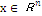represent the average travel time on each link of the network. It is the variable that we try to estimate. For a vehicle, with a path represented by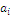and the travel time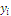, we have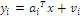where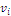represents some noise in the measurements.

## b

The ordinary least-squares (OLS) problem is a particularly simple optimization problem that involves the minimization of the Euclidean norm of a ‘‘residual error" vector that is affine in the decision variables. Given n measurements of path travel times, we would like to estimate the average travel time on each link of the network using least-square estimation:where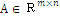,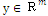are given. The combination of A, y: (A,y) is often referred to as the problem data. The solution of the above optimization problem is given by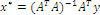if the matrix A is full column rank.